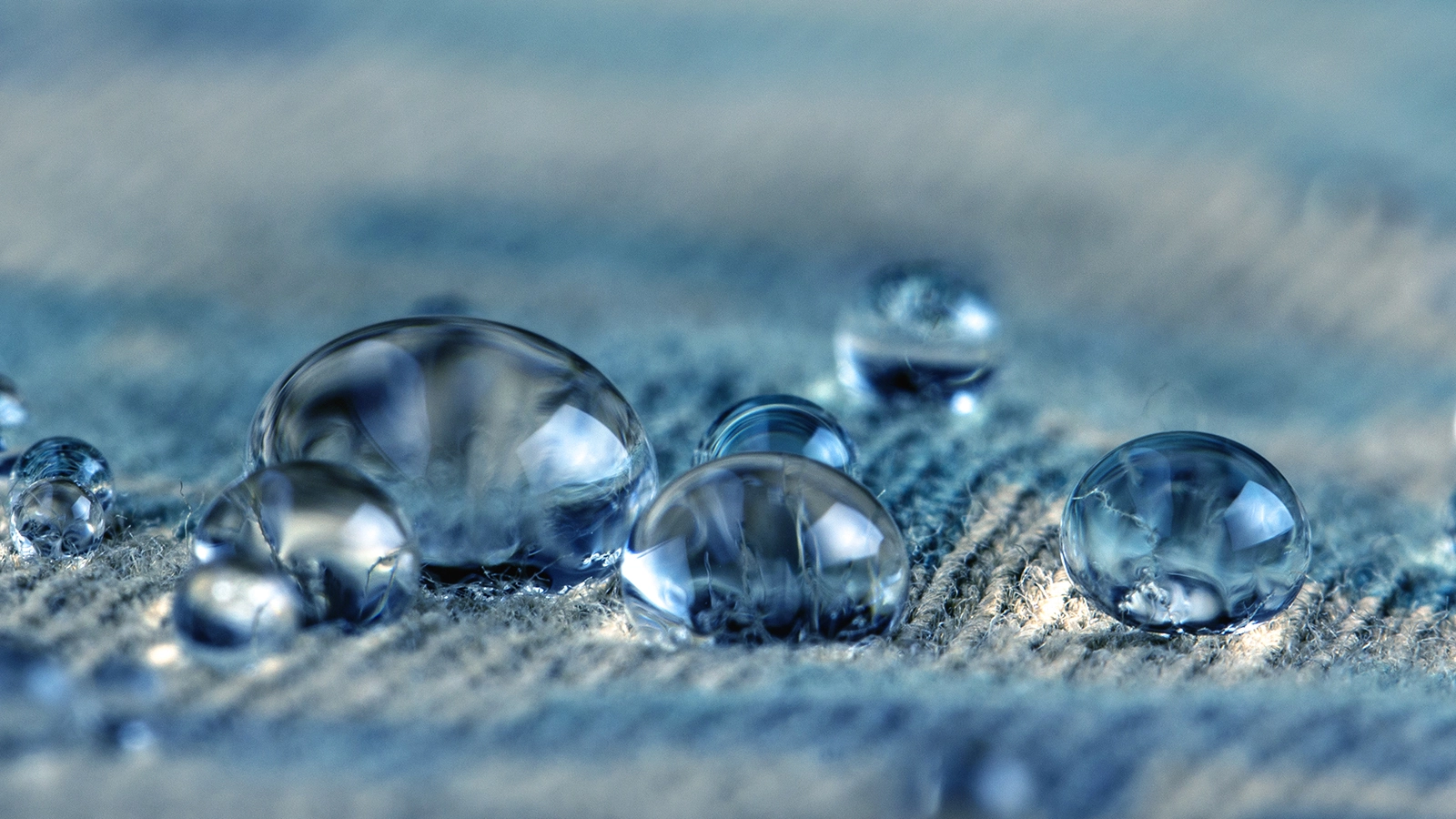# Contact Angle Measurement

Contact angle measurements are typically done by using either optical or force tensiometers. Contact angles can be divided into static, dynamic, and roughness corrected contact angles. Contact angle hysteresis and roll-off angles are also measured.

## What is Contact Angle?

Contact angle, θ (theta), is a quantitative measure of wetting of a solid by a liquid. The contact angle is geometrically defined as the angle formed by a liquid at the three-phase boundary where a liquid, gas, and solid intersect. There are three different forces acting on this three-phase contact point between solid, liquid and gas, or in some cases solid, liquid and liquid. Contact angles can be divided into static, dynamic and roughness corrected contact angle.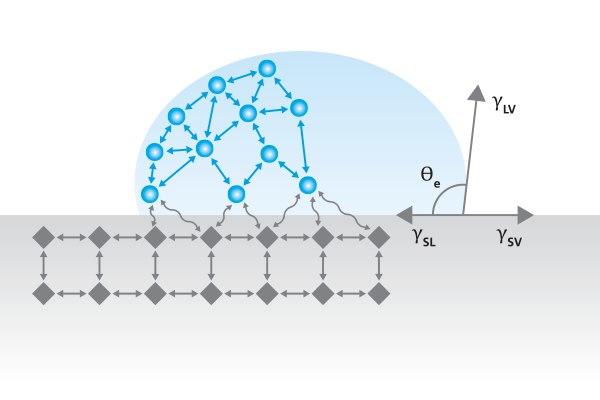### Static contact angle

Static (i.e. sessile drop) contact angles are measured when the droplet is sitting on the surface and the three-phase boundary is not moving. Static contact angles are by far the most measured wettability values. It is most suitable for relatively smooth and homogenous surfaces. Static contact angles are also used to define the surface free energy (i.e. surface tension of solid) of the substrate. Static contact angle offers a quick, easy and quantitative measurement of wettability. Static contact angle measurement is based on Young’s equation which assumes that interfacial forces are thermodynamically stable.

### Dynamic contact angle, contact angle hysteresis and roll-off angle

When the three-phase boundary is moving, dynamic contact angles can be measured, and are referred to as advancing and receding angles. As the terms imply, advancing contact angle is measured when the droplet front is advancing and receding when the droplet front is receding. On an ideal surface, these two values are close to each other. However, most often, the measured contact angle depends on the direction on which the contact line is moving. Contact angle hysteresis is the difference between the advancing and the receding contact angles. Contact angle hysteresis arises mostly from the chemical and topographical heterogeneity of the surface, solution impurities absorbing on the surface, or swelling, rearrangement or alteration of the surface by the solvent.

The roll-off angle refers to a tilting angle at which the droplet starts to move. A low roll of angle is related to low contact angle hysteresis.

### Roughness corrected contact angles

Contact angles are affected by roughness. Surfaces with the same chemical composition but different roughness will have different contact angles. Roughness corrected contact angles are contact angle values where the effect of roughness is taken away. Roughness corrected contact angles should especially be used when surface free energies are determined.## Optical Tensiometer for Contact Angle Measurement

Optical tensiometers are utilized to measure static, dynamic and roughness corrected contact angles. The main components of the optical tensiometer are a camera, dispenser to dispense a drop, sample stage and the light source to illuminate the drop on the sample stage. Optical tensiometers range from completely manual systems to fully automated instruments.

Optical tensiometer is sometimes also called optical or contact angle goniometer, contact angle meter or drop shape analyzer. Essentially all of these mean the similar type of instrument which we use the name optical tensiometer.

### Static contact angle with optical tensiometer

Sessile drop measurement is the most commonly used contact angle measurement method. In practice, a droplet is placed on the solid surface and an image of the drop is recorded. The static contact angle is then defined by fitting Young-Laplace equation around the droplet, although other fitting methods such as circle and polynomial can also be used.

It is also possible to measure inverted sessile drops called captive bubbles. The captive bubble measurements are especially utilized to study wettability of the contact lens. It is also suitable for other highly hydrophilic materials where the standard sessile drop method is challenging. Also, it is very much used in applications where the contact angles of immersed objects are being measured. For example, measurement of oil drop on a rock surface immersed in water.### Dynamic contact angle with optical tensiometer

Dynamic contact angle measurements with the optical tensiometer are done with two main methods: needle method and tilting method.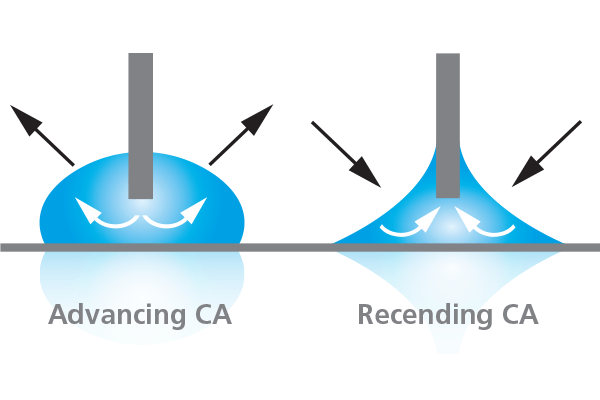In the needle method, a small droplet is first formed and placed on the surface. The needle is then brought close to the surface and the volume of the droplet is gradually increased while recording the contact angle at the same time. This will give the advancing contact angle. The receding angle is measured the same way but this time, the volume of the droplet is gradually decreased. This method is especially utilized to measure dynamic contact angles on superhydrophobic surfaces.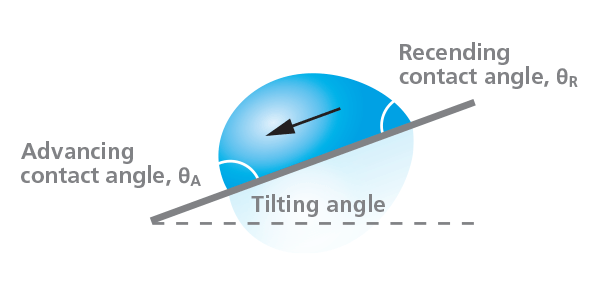In the tilting method, the droplet is placed on the substrate which is then gradually tilted. The advancing angle is measured at the front of the droplet just as the droplet starts to move. The receding contact angle is measured at the back of the droplet, at the same time point. With this method, the roll-off or sliding angle can also be determined. The roll-off angle refers to a tilting angle at which the droplet starts to move. A low roll of angle is related to low contact angle hysteresis. The tilting can be done either by tilting the sample stage or by tilting the whole instrument.### Roughness corrected contact angle with optical tensiometer

Roughness corrected contact angles are measured with the combined surface roughness and contact angle measurements. The optical tensiometer is combined with the topography module to facilitate the roughness measurements. The roughness is measured at the same spot as the contact angle.

The roughness ratio is used to correct the contact angle for surface roughness. The roughness ratio is defined as the ratio between the actual and projected solid surface area (r = 1 for a smooth surface and > 1 for a rough surface). Wenzel equation explains the relation between roughness ratio and measured and corrected contact angle. It is important to note that the Wenzel equation assumes that the liquid completely penetrates into the roughness grooves.## Force Tensiometer for Contact Angle Measurement

Force tensiometry consists of a highly sensitive balance where the sample is attached through a hook. The test liquid is placed on a measurement cup on the sample stage that can be moved either manually or automatically. Force tensiometers range from completely manual stand-alone systems to fully automated computer-controlled instruments. For contact angle measurements the fully automated instruments are used.

With the force tensiometer, the measured contact angles are always advancing and receding angles and contact angle hysteresis. Due to dynamic nature of the method it is not possible to measure static contact angles.

### Wilhelmy plate method

The contact angle measurement with the force tensiometer is based on so-called Wilhelmy plate method. Force tensiometer measures the mass affecting to the balance when a solid sample is brought in contact with the test liquid. The contact angle can then be calculated when the surface tension of the liquid and the perimeter of the sample are known. The advancing angle is measured as the sample is immersed into the liquid and receding angle when the sample is withdrawn out from the liquid. As the perimeter of the sample must be known, the sample has to be rectangular or rod shape. The contact angle is averaged across the whole area dipped into the test liquid. This means that the surface has to be the same on both sides and the edges of the sample. Wilhelmy plate is then not suitable for the contact angle measurements of surface treatments done just one side of the sample.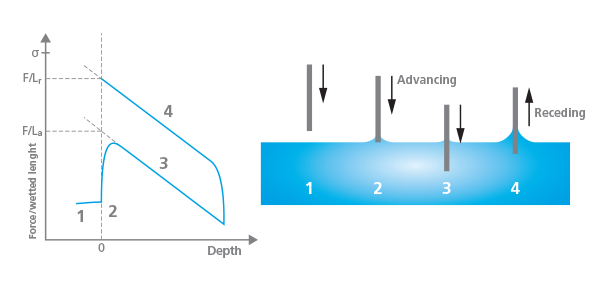## Contact Angle Measurements on Special Samples

As contact angle measurements are found useful in many industrial areas, there is an increasing need to measure them also on more unconventional samples such as fibers, powders, and on small areas where a standard microliter size drop doesn’t fit.

### Contact angle measurements on fibers

Fibers and other thin objects can be measured by using optical tensiometer equipped with picoliter dispenser, with the meniscus method or with force tensiometer using Wilhelmy method. With the optical tensiometer, the picoliter dispenser can produce droplets with the diameter of about 30 μm in air. With special optics and a high-speed camera, it is possible to take an image of this small droplet and the contact angle can be determined in a similar fashion than by using microliter size droplets.

Meniscus measurement can also be used for fibers. In this method, the sample is first immersed into liquid. When the sample is pulled from the liquid a meniscus is formed and the contact angle is optically measured. If the fiber is thin (below 200 μm) a special optics is required.

Force tensiometer, on the other hand, can be used by utilizing a special holder for the fiber. Down to 7 μm, fibers can be measured. It should be noted that the measurement with picoliter droplets leads to static contact angles whereas the Wilhelmy method is dynamic, giving both advancing and receding angles. The contact angle measured with the meniscus method is somewhere in between and cannot be directly compared with contact angles measured with the other two methods.

### Contact angle measurements on powders

Contact angles on powders can be measured by using sessile drop measurement on the compressed powder tablet or by using the force tensiometer with the Washburn method. When compressed tablets are measured, it can behave like the absorbing substrate if the powder is hydrophilic or the droplet can stay on the surface if the powder is hydrophobic. The main issue with the tablet compression is that the surface properties of the tablets can vary a lot, which can lead to misinterpretation of the results. Roughness on the tablet surface could be considered by measuring the roughness corrected contact angle.

To study loose powders, the Washburn method must be utilized. In this method, the powder is packed in a cylinder with holes at the bottom. The holder is slightly immersed into measure­ment liquid and the liquid uptake as a function of time is recorded. The time it takes for the liquid to penetrate is related to contact angle through Washburn equation. As the method is based on the capillary action, it is limited to below 90° contact angles.### Contact angle measurements on small areas

In some cases, the contact angle measurements need to be done on small areas. This could be anything from a printed circuit board to a catheter surface. For these measurements, picoliter-sized drops are needed. The measurements can be done with the optical tensiometer equipped with a picoliter dispenser that produces small enough drops to fit on the location measured. High magnification optic is required for drop visualization. Apart from that, the measurement is similar to the standard static contact angle measurement.

## Contact Angle Measurements at Extreme Conditions

Contact angle measurements are most commonly done at ambient conditions but in some cases, temperatures and even pressures need to be controlled.

For temperature control, there are cooling and heating options for both optical and force tensiometer. Cooling and heating are most typically done with circulators that can control the temperature on wide range from below zero to 150 °C. If even higher temperatures are needed, the optical tensiometer can be equipped with electrically heated chamber that goes up to 250 °C.

For measurements at high pressures, a high-pressure tensiometer is required. In addition to the standard camera and light source needed for contact angle measurements, the high-pressure tensiometer includes a high-pressure chamber. The instrument allows contact angle measurements up to 400 bar and the temperature range from 1 to 200 °C. The high pressure tensiometer is especially utilized to study wettability at reservoir conditions for enhanced oil recovery optimization.## Standards for Contact Angle Measurements

There are several different standards for contact angle measurements with optical tensiometers. Standards are mainly related to very practical applications such as testing of surface cleanliness and evaluation of surface after plasma or corona treatment. There are also standards for surface free energy determination through contact angle measurements and more general guidelines for how to perform dynamic contact angle measurements with the needle or tilt method.

## Related products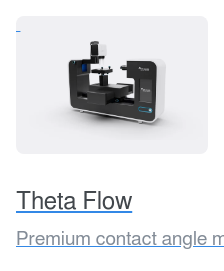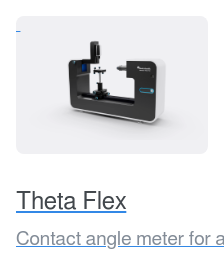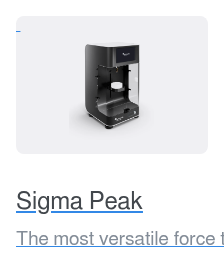#### Save it for later?

We have put all the knowledge of this page in a guide for you to read when you have the time.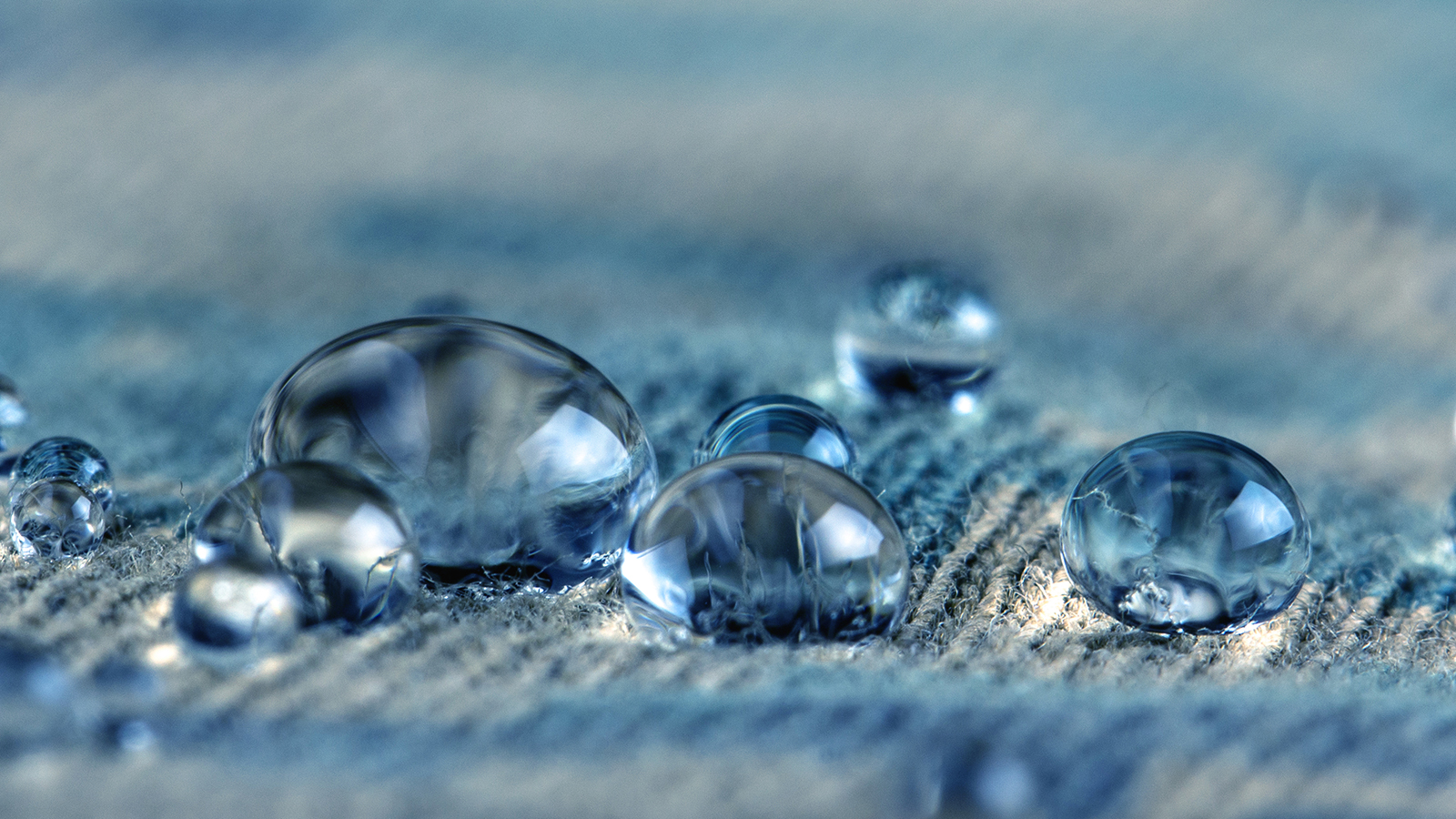## Save it for later?

We have put all the knowledge of this page in a guide for you to read when you have the time.